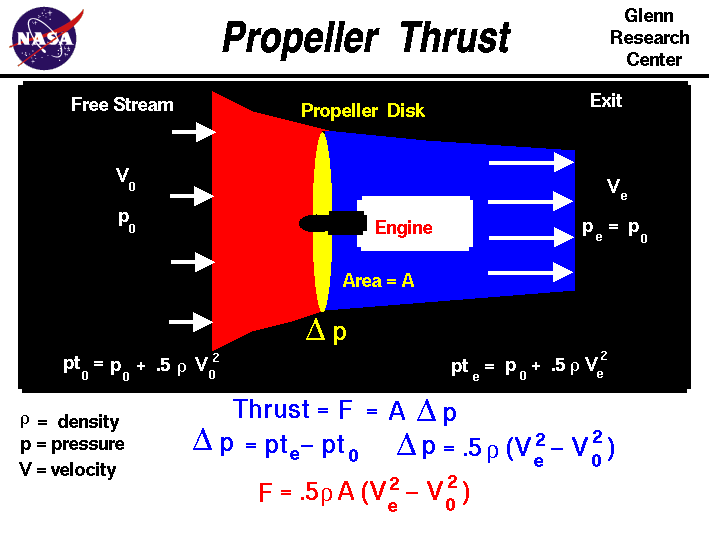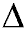+ Text Only Site
+ Non-Flash Version
+ Contact GlennMost general aviation or private airplanes are powered by internal combustion engines which turn propellers to generate thrust. The details of how a propeller generates thrust is very complex, but we can still learn a few of the fundamentals using the simplified momentum theory presented here. Propeller Propulsion System On the slide, we show a schematic of a propeller propulsion system at the top and some of the equations that define how a propeller produces thrust at the bottom. The details of propeller propulsion are very complex because the propeller is like a rotating wing. Propellers usually have between 2 and 6 blades. The blades are usually long and thin, and a cut through the blade perpendicular to the long dimension will give an airfoil shape. Because the blades rotate, the tip moves faster than the hub. So to make the propeller efficient, the blades are usually twisted. The angle of attack of the airfoils at the tip is lower than at the hub because it is moving at a higher velocity than the hub. Of course, these variations make analyzing the airflow through the propeller a verydifficult task. Leaving the details to the aerodynamicists, let us assume that the spinning propeller acts like a disk through which the surrounding air passes (the yellow ellipse in the schematic). The engine, shown in white, turns the propeller and does work on the airflow. So there is an abrupt change in pressure across the propeller disk. (Mathematicians denote a change by the Greek symbol "delta" (). Across the propeller plane, the pressure changes by "delta p" (p). The propeller acts like a rotating wing. From airfoil theory, we know that the pressure over the top of a lifting wing is lower than the pressure below the wing. A spinning propeller sets up a pressure lower than free stream in front of the propeller and higher than free stream behind the propeller. Downstream of the disk the pressure eventually returns to free stream conditions. But at the exit, the velocity is greater than free stream because the propeller does work on the airflow. We can apply Bernoulli'sequation to the air in front of the propeller and to the air behind the propeller. But we cannot apply Bernoulli's equation across the propeller disk because the work performed by the engine violates an assumption used to derive the equation. Simple Momentum Theory Turning to the math, the thrust F generated by the propeller disk is equal to the pressure jump delta p times the propeller disk area A: F = delta p * A A units check shows that: force = (force/area) * area We can use Bernoulli's equation to relate the pressure and velocity ahead of and behind the propeller disk, but not through the disk. Ahead of the disk the total pressure pt0 equals the static pressure p0 plus the dynamic pressure .5 * r * V0 ^2. pt0 = p0 + .5 * r * V0 ^2 where r is the air density and V0 is the velocity of the aircraft. Downstream of the disk, pte = p0 + .5 * r * Ve ^2 where pte is the downstream total pressure and Ve is the exit velocity. At the disk itself the pressure jumps delta p = pte - pt0 Therefore, at the disk, delta p = .5 * r * [Ve ^2 - V0 ^2] Substituting the values given by Bernoulli's equation into the thrust equation, we obtain F = .5 * r * A * [Ve ^2 - V0 ^2] We still must determine the magnitude of the exit velocity. A propeller analysis based on the momentum equation provides this value. Note that this thrust is an ideal number that does not account for many losses that occur in practical, high speed propellers, like tip losses. The losses must be determined by a more detailed propeller theory, which is beyond the scope of these pages. The complex theory also provides the magnitude of the pressure jump for a given geometry. The simple momentum theory, however, provides a good first cut at the answer and could be used for a preliminary design. Activities: Guided ToursPropellers:Turboprops:Navigation ..Beginner's Guide Home Page+ Inspector General Hotline + Equal Employment Opportunity Data Posted Pursuant to the No Fear Act + Budgets, Strategic Plans and Accountability Reports + Freedom of Information Act + The President's Management Agenda + NASA Privacy Statement, Disclaimer, and Accessibility CertificationEditor: Nancy Hall NASA Official: Nancy Hall Last Updated: May 13 2021 + Contact Glenn